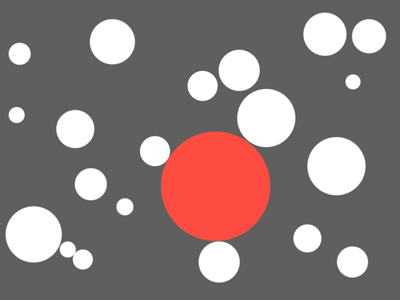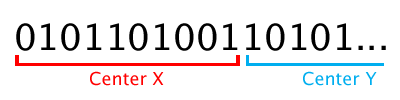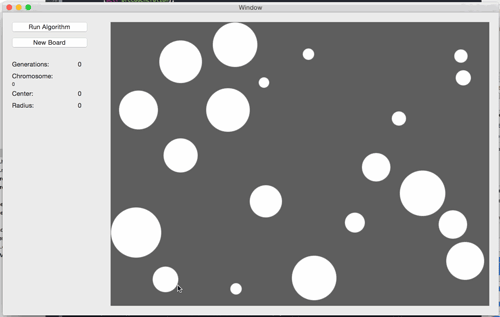# Writing a Basic Genetic Algorithm in Obj-CAfter hearing a lot about genetic algorithms recently, I wanted to get stuck in myself and learn how it all works. Especially after reading Roger Alsing’s recent post on the Evolution of Mona Lisa (which is totally awesome).

Given a space with a number of non-overlapping circles, find the largest circle that can fit without overlapping any of the others

Like this:# The Basics

A genetic algorithm (GA) is a surprisingly simple idea at its core. In fact, of the few programs I’ve built so far, a lot of the code is exactly the same.

The algorithm I’ll discuss here works as follows:

1. Generate a random population of possible solutions
2. Assign a fitness score to each solution in the population
3. “Breed” a new generation of solutions by selecting random pairs (weighted by their fitness score) from the old population
4. Crossover each selected pair according to a crossover rate
5. Mutate each pair according to a mutation rate
6. Repeat steps 2 through 5

# Implementation

### Chromosomes and Genes

First up, we need a representation of our solution. We call this a chromosome.

Given that we want to find a circle, our solution needs to provide a position and a radius. We’ll encode these values in a binary string.

To store these values, we’ll divide our chromosome into three parts (or genes): center-x, center-y, and radius. If we use 10 bits for each gene, it looks like this:To make reading out the encoded data a little easier, we’ll wrap this up in a class called `LargestCircleChromosome`. This class decodes the chromosome string as soon as it’s assigned, and stores the values in a `Circle` object.

``````static NSInteger const kGeneLength = 10;
static NSInteger const kChromosomeLength = 3 * kGeneLength;``````
``````- (void)setChromosomeString:(NSString *)chromosomeString {
_chromosomeString = chromosomeString;

NSString *centerXGene = [self.chromosomeString substringWithRange:NSMakeRange(0, kGeneLength)];
NSNumber *centerX = [self numberFromBinaryString:centerXGene];
NSString *centerYGene = [self.chromosomeString substringWithRange:NSMakeRange(kGeneLength, kGeneLength)];
NSNumber *centerY = [self numberFromBinaryString:centerYGene];

_circle = [[Circle alloc] init];
_circle.position = CGPointMake([centerX floatValue], [centerY floatValue]);

NSString *radiusGene = [self.chromosomeString substringWithRange:NSMakeRange(kGeneLength * 2, kGeneLength)];
}``````

### Fitness Score

Next, we need a way to measure the fitness score for a chromosome. This is the hardest part to get right, and how you implement it will vary depending on the problem you’re trying to solve.

The fitness score needs to be able to indicate how much better one chromosome is than another. The following method is effective enough for solving our problem:

``````- (CGFloat)fitnessScoreForChromosome:(LargestCircleChromosome *)chromosome {

CGRect intersectWithBoard = CGRectIntersection(circleRect, CGRectMake(0.f, 0.f, kBoardSize.width, kBoardSize.height));

// Out of bounds
if (CGRectEqualToRect(intersectWithBoard, circleRect) == NO) return 0.f;

// Overlaps with another circle
NSUInteger numberOfOverlaps = [self.board numberOfOverlapsForCircle:chromosome.circle];
if (numberOfOverlaps > 0) return 0.f;

}``````

A few things to note here:

• If the circle goes out of bounds at all, give it a fitness score of 0 (effectively killing it off in this generation)
• If the circle overlaps with any other circle, also kill it off with a fitness score of 0
• The return value could simply be the radius, however using an exponent will cause the GA to favor slightly larger radii a lot better, and help us get an answer much faster

### Roulette Wheel Selection

Selecting random chromosomes according to their fitness score is also referred to as “roulette wheel selection”. You can imagine dividing up a circle into slices where the fitness score determines the size of each slice, then spinning it to choose our random chromosome.

Since we’re pulling out pairs, we can write this as a recursive method to pull out any number of chromosomes.

``````- (NSArray *)selectWeightedRandomChromosomes:(NSUInteger)numberToSelect {

NSMutableArray *chromosomes = [NSMutableArray array];
if (numberToSelect > 1) {
}

NSArray *remainingPopulation = [self.population arrayByRemovingObjectsFromArray:chromosomes];
__block CGFloat totalFitness = 0;
[remainingPopulation enumerateObjectsUsingBlock:^(LargestCircleChromosome *chromosome, NSUInteger idx, BOOL *stop) {
totalFitness += chromosome.fitness;
}];
CGFloat selectedFitness = drand48() * totalFitness;

__block CGFloat iteratingFitness = 0.f;
[remainingPopulation enumerateObjectsUsingBlock:^(LargestCircleChromosome *chromosome, NSUInteger idx, BOOL *stop) {
iteratingFitness += chromosome.fitness;
if (iteratingFitness >= selectedFitness) {
*stop = YES;
}
}];

return chromosomes;
}``````

### Crossover and Mutation

The last building block we need is a way to crossover and mutate our chromosomes.

We start by defining a crossover rate and mutation rate. Playing with these values is very important to get a feel for how it affects the efficiency of the algorithm. The crossover rate can be quite high - we’ll use `0.7` - while the mutation rate should stay very low - we’ll use `0.01`. I played with mutation rates ranging from `0.001` through to `0.1` which had varying effects on how the program performed.

``````static CGFloat const kCrossOverRate = 0.7;
static CGFloat const kMutationRate = 0.01;``````

Crossing over a pair of chromosomes is done by selecting a random point along both binary strings, and swapping the second section of each string:Which, in code, looks like this:

``````- (NSArray *)crossOverChromosome:(LargestCircleChromosome *)chromosomeOne withCromosome:(LargestCircleChromosome *)chromosomeTwo {

NSUInteger splitPoint = arc4random_uniform((UInt32)chromosomeOne.chromosomeString.length);

NSMutableString *chromosomeStringOne = [[NSMutableString alloc] init];
[chromosomeStringOne appendString:[chromosomeOne.chromosomeString substringWithRange:NSMakeRange(0, splitPoint)]];
[chromosomeStringOne appendString:[chromosomeTwo.chromosomeString substringWithRange:NSMakeRange(splitPoint, chromosomeTwo.chromosomeString.length - splitPoint)]];

NSMutableString *chromosomeStringTwo = [[NSMutableString alloc] init];
[chromosomeStringTwo appendString:[chromosomeOne.chromosomeString substringWithRange:NSMakeRange(splitPoint, chromosomeOne.chromosomeString.length - splitPoint)]];
[chromosomeStringTwo appendString:[chromosomeTwo.chromosomeString substringWithRange:NSMakeRange(0, splitPoint)]];

[self mutateChromosomeString:chromosomeStringOne];
[self mutateChromosomeString:chromosomeStringTwo];

return @[[LargestCircleChromosome chromosomeWithString:chromosomeStringOne], [LargestCircleChromosome chromosomeWithString:chromosomeStringTwo]];
}``````

You’ll notice the two calls to the `mutateChromosomeString:` method. As part of the crossover process, we apply our mutation function to each new chromosome.

Mutation is accomplished by looping through each bit in the chromosome binary string, and randomly toggling the value of each bit according to our mutation rate (defined above as `0.01`).

``````- (void)mutateChromosomeString:(NSMutableString *)chromosomeString {
for (NSUInteger i = 0; i < chromosomeString.length; i++) {
if (drand48() > kMutationRate) continue;
NSString *currentValue = [chromosomeString substringWithRange:NSMakeRange(i, 1)];
[chromosomeString replaceCharactersInRange:NSMakeRange(i, 1) withString:[currentValue isEqualToString:@"0"] ? @"1" : @"0"];
}
}``````

# Putting It All Together

Now we can put all these parts together to perform the algorithm outlined at the beginning.

``````- (void)runWithCompletion:(void(^)(AlgorithmResult *result))completion progress:(void(^)(AlgorithmResult *result))progress {
self.isRunning = YES;

dispatch_async(dispatch_get_global_queue(DISPATCH_QUEUE_PRIORITY_DEFAULT, 0), ^{

[self generateRandomPopulation];

__block NSUInteger generationCount = 0;

while (self.isRunning == YES) {
[self.population enumerateObjectsUsingBlock:^(LargestCircleChromosome *chromosome, NSUInteger idx, BOOL *stop) {
chromosome.fitness = [self fitnessScoreForChromosome:chromosome];
}];
generationCount++;

dispatch_async(dispatch_get_main_queue(), ^{
AlgorithmResult *algorithmProgress = [AlgorithmResult resultWithChromosome:[self fittestChromosome] generations:generationCount];
progress(algorithmProgress);
});

[self breedGeneration];
}

dispatch_async(dispatch_get_main_queue(), ^{
self.isRunning = NO;

AlgorithmResult *algorithmResult = [AlgorithmResult resultWithChromosome:[self fittestChromosome] generations:generationCount];
completion(algorithmResult);
});
});
}

- (void)stop {
self.isRunning = NO;
}

- (void)breedGeneration {
NSMutableArray *newPopulation = [NSMutableArray array];
while (newPopulation.count < kPopulationSize) {

NSArray *chromosomePair = [self selectWeightedRandomChromosomes:2];

NSArray *newChromosomePair;
if (drand48() < kCrossOverRate && chromosomePair.count > 1) {
newChromosomePair = [self crossOverChromosome:chromosomePair withCromosome:chromosomePair];
} else {
newChromosomePair = chromosomePair;
}

Dispatching this algorithm on a background thread lets us view the progress as it improves its best answer. We can use the `progress()` and `completion()` blocks to update our UI to reflect the current state of the system.